RRB JE CBT 1 - Full Test 6

# RRB JE CBT 1 - Full Test 6

Test Description

## 100 Questions MCQ Test RRB JE (Railways Junior Engineer) CBT Mock Test Series | RRB JE CBT 1 - Full Test 6

RRB JE CBT 1 - Full Test 6 for Railways 2023 is part of RRB JE (Railways Junior Engineer) CBT Mock Test Series preparation. The RRB JE CBT 1 - Full Test 6 questions and answers have been prepared according to the Railways exam syllabus.The RRB JE CBT 1 - Full Test 6 MCQs are made for Railways 2023 Exam. Find important definitions, questions, notes, meanings, examples, exercises, MCQs and online tests for RRB JE CBT 1 - Full Test 6 below.
Solutions of RRB JE CBT 1 - Full Test 6 questions in English are available as part of our RRB JE (Railways Junior Engineer) CBT Mock Test Series for Railways & RRB JE CBT 1 - Full Test 6 solutions in Hindi for RRB JE (Railways Junior Engineer) CBT Mock Test Series course. Download more important topics, notes, lectures and mock test series for Railways Exam by signing up for free. Attempt RRB JE CBT 1 - Full Test 6 | 100 questions in 90 minutes | Mock test for Railways preparation | Free important questions MCQ to study RRB JE (Railways Junior Engineer) CBT Mock Test Series for Railways Exam | Download free PDF with solutions
 1 Crore+ students have signed up on EduRev. Have you?
RRB JE CBT 1 - Full Test 6 - Question 1

### Weight of Alok is twice the weight of Karan. Weight of Karan is one-fourth of the weight of Roma. Weight of Roma is twice of the weight of Tipu. Weight of Tipu is twice of the weight of Shivam. The weight of Roma is greater than the weight of how many persons among Alok, Karan, Shivam and Tipu?

Detailed Solution for RRB JE CBT 1 - Full Test 6 - Question 1

Let weight of Shivam be ‘x’.

Weight of Tipu is twice of the weight of Shivam: 2x,

Weight of Roma is twice of the weight of Tipu: 4x,

Weight of Karan is one-fourth of the weight of Roma: ‘x’,

Weight of Alok is twice the weight of Karan: 2x

Hence, weight of Roma is greater than all other persons.

Hence, 4 is the correct answer.

RRB JE CBT 1 - Full Test 6 - Question 2

### In the following question, select the odd image from the given alternatives.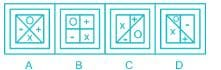Detailed Solution for RRB JE CBT 1 - Full Test 6 - Question 2

Here in all the figure, O is vertically opposite to X and + is vertically opposite to -, except figure D

Hence, Fig: -D is the odd image among the given figure.

RRB JE CBT 1 - Full Test 6 - Question 3

### Vijay Diwas is celebrated every year in India on which day?

Detailed Solution for RRB JE CBT 1 - Full Test 6 - Question 3
• Vijay Diwas is celebrated every year in India on 16 December.
• The day marks the Indian military's victory over Pakistan in 1971 during the war for the independence of Bangladesh from Pakistan.
• On this day, 93,000 members of Pakistani troops surrendered to the Indian Army and Mukti Bahini, calling an end to the Indo-Pak war of 1971.
• Kargil Vijay Diwas, named after the success of Operation Vijay.
• On this day, 26 July 1999, India successfully took command of the high outposts which had been lost to Pakistani intruders.
RRB JE CBT 1 - Full Test 6 - Question 4

A piece of paper is folded and punched as shown in the question figure. From the given answer figures, indicate how it will appear when opened?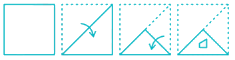Detailed Solution for RRB JE CBT 1 - Full Test 6 - Question 4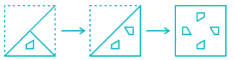The final figure will be as shown above.

RRB JE CBT 1 - Full Test 6 - Question 5

A garrison is provided with ration for 60 soldiers to last for 72 days. Find out how many days they will last if soldiers are 40 and they increase their intake by 20%?

Detailed Solution for RRB JE CBT 1 - Full Test 6 - Question 5

It is a case of indirect proportion

More men, Less days

Number of days = (60 × 72)/(40 × 120/100) = 90 days

RRB JE CBT 1 - Full Test 6 - Question 6

In the following question, select the related number from the given alternatives.
54 : 135 ∷ 32 : ?

Detailed Solution for RRB JE CBT 1 - Full Test 6 - Question 6

The logic followed here is:

54 : 135 → (27 × 2 = 54; 27 × 5 = 135)

32 : ? → (16 × 2 = 32; 16 × 5 = 80)

RRB JE CBT 1 - Full Test 6 - Question 7

The compound interest on a certain sum for 2 years is Rs. 41 and the simple interest is Rs. 40. Find the rate % p.a.

Detailed Solution for RRB JE CBT 1 - Full Test 6 - Question 7

Let the sum be ‘x’ and the rate be ‘r’
∴ According to the 1st given condition,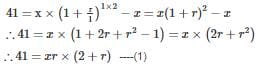According to the 2nd given condition,

⇒ 40 = (x × 2 × r)

∴ xr = 20

Substituting in equation (1) we get,

⇒ 41 = 20 × (2 + r)

∴ r = 0.05

∴ The required rate is 5%

RRB JE CBT 1 - Full Test 6 - Question 8

Who among the following discovered the structure of an atom?

Detailed Solution for RRB JE CBT 1 - Full Test 6 - Question 8

Ernest Rutherford and Niels Bohr discovered the structure of an atom.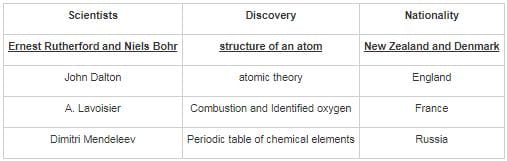RRB JE CBT 1 - Full Test 6 - Question 9

The number of males per hundred females in a country was 120. At the next census, the total population increased by 5% and the female population increased by 10%. Find the increase percent in the male population.

Detailed Solution for RRB JE CBT 1 - Full Test 6 - Question 9

Given, the number of males per hundred females in a country was 120.

Let the number of females be 100a, thus the number of males will be 120a.

Given, at the next census, the total population increased by 5% and the female population increased by 10%.

Let the % increase in the population be ‘b%’

∴ b% of 120a + 10% of 100a = 5% of 220a

⇒ 1.2b + 10 = 11

⇒ b = 1/1.2 = 5/6% = 0.8333%

RRB JE CBT 1 - Full Test 6 - Question 10

Direction: In the question below are given three statements followed by three conclusions numbered I, II and III. You have to take the given statements to be true even if they seem to be at variance with commonly known facts. Read all the conclusions and then decide which of the given conclusions logically follows from the given statements disregarding commonly known facts.

Statements:
Some actors are not a singer
No singer is a dancer
All artist is a singer

Conclusions:
I. All dancers are an artist
II. Some artist are not actors
III. Some actors are dancers is a possibility

Detailed Solution for RRB JE CBT 1 - Full Test 6 - Question 10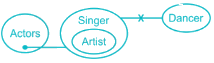Conclusions:

I. All dancers are artist → False (As, No singer is dancer and All artist are singer)

II. Some artists are not actors → False (As, Some actors are not singer and All artist are singer → It can be true but it is not definite)

III. Some actors are dancers is a possibility → True (As, Some actors are not singer and No singer is dancer → It can be true)Hence, only conclusion III follows.

RRB JE CBT 1 - Full Test 6 - Question 11

A man goes to a place at a speed of 20 km/hr and comes back with a different speed. If the average speed is 8 km/hr, then what is the return speed (in km/hr)?

Detailed Solution for RRB JE CBT 1 - Full Test 6 - Question 11

We know that,

Average Speed = Total distance/Total time

⇒ Average speed =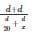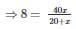⇒ x = 5 km/hr

RRB JE CBT 1 - Full Test 6 - Question 12

Three men, four women and six children can complete a work in seven days. A woman does double the work a man does and a child does half the work a man does. How many women alone can complete this work in 7 days?

Detailed Solution for RRB JE CBT 1 - Full Test 6 - Question 12

Let 1 woman’s 1 day’s work = x

⇒ 1 man’s 1 day’s work = x/2 and 1 child’s 1 day’s work = x/4

⇒ (3x/2 + 4x + 6x/4) = 1/7

⇒ 28x/4 = 1/7

⇒ x = (1/7 × 4/28) = 1/49

⇒ 1 woman can complete the work in 49 days.

∴ To complete the work in 7 days, number of women required = (49/7) = 7

RRB JE CBT 1 - Full Test 6 - Question 13

What will come in place of question mark (?) in the given equation?
14 ? 8 ? 16 ? 4 = 16

Detailed Solution for RRB JE CBT 1 - Full Test 6 - Question 13

Given Expression: 14 ? 8 ? 16 ? 4 = 16

Let us check each option;

1) +, ÷ and –

14 + 8 ÷ 16 – 4 = 16

14 + 0.5 – 4 = 10.5

Hence, it is false.

2) ×, + and –

14 × 8 + 16 – 4 = 16

112 + 16 – 4 = 124

Hence, it is false.

3) ÷, + and ×

14 ÷ 8 + 16 × 4 = 16

1.75 + 64 = 65.75

Hence, it is false.

4) +, ÷ and ×

14 + 8 ÷ 16 × 4 = 16

14 + 0.5 × 4

14 + 2 = 16

Clearly, it is true.

Hence, +, ÷ and × will come in place of question mark.

RRB JE CBT 1 - Full Test 6 - Question 14

Arrangement of leaves in a plant is called as ________.

Detailed Solution for RRB JE CBT 1 - Full Test 6 - Question 14

The arrangement of leaves on a stem is known as phyllotaxy. Leaves can be classified as either alternate, spiral, opposite, or whorled.

RRB JE CBT 1 - Full Test 6 - Question 15

The students in three classes are in the ratio 4 : 6 : 9. If 12 students are increased in each class, the ratio changes to 7 : 9 : 12. Then the total number of students in the 2nd Class before the increase is

Detailed Solution for RRB JE CBT 1 - Full Test 6 - Question 15

The Initial ratio is 4 : 6 : 9

Let the common ratio be ‘x’

Therefore the respective number of students in the 3 classes will be 4x , 6x and 9x

After addition of 12 students to each class,

The numbers will be 4x + 12 , 6x + 12, 9x + 12

The ratio after that will be 7 : 9 : 12

∴ (4x + 12)/(6x + 12) = 7/9

∴ 9 × (4x + 12) = 7 × (6x + 12)

∴ 36x + 108 = 42x + 84

∴ 6x = 24

∴ x = 4

∴ The initial number of students in the 2nd class = 24

RRB JE CBT 1 - Full Test 6 - Question 16

Which part of the brain is responsible for the feeling of thirst, hunger and sleep?

Detailed Solution for RRB JE CBT 1 - Full Test 6 - Question 16
• Hypothalamus is a section of the brain which is responsible for hormone production.
• The hormones produced by this area of the brain governs body temperature, thirst, hunger, sleep and mood.
RRB JE CBT 1 - Full Test 6 - Question 17

Which metal is common to the alloys brass, bronze, and gunmetal?

Detailed Solution for RRB JE CBT 1 - Full Test 6 - Question 17

Modified gunmetalt is typically composed of 86% copper, 9.5% tin, 2.5% lead, and 2% zinc. Brass is an alloy of copper and zinc, while bronze is an alloy consisting mainly of copper, combined most often with tin.

RRB JE CBT 1 - Full Test 6 - Question 18

Which of the following is related to the Second Schedule of the Indian Constitution?

Detailed Solution for RRB JE CBT 1 - Full Test 6 - Question 18
• Second schedule lists the emoluments for holders of constitutional offices such as salaries of President, Vice President, Ministers, Judges and Comptroller and Auditor-General of India etc.
• Representation in the RS is defined in the Fourth Schedule whereas Languages are defined in the Eighth Schedule.
• The aspects of oath taking have been taken care of in the Third Schedule of the Constitution.
RRB JE CBT 1 - Full Test 6 - Question 19

Directions:  One statement is given, followed by two conclusions, I and II. You have to consider the statement to be true, even if it seems to be at variance from commonly known facts. You are to decide which of the given conclusions be definitely drawn from the given statement.

Statement: Admissions in all departments are closed since no more seats are vacant and students are actively involved in their academics.

Conclusions:
I.
Students prefer to study any subject of their interest.
II. Importance of education is known to the students.

Detailed Solution for RRB JE CBT 1 - Full Test 6 - Question 19

Solution: Since the seats in every department are filled without any vacancy it is known that the interest of every student varies and they tend to study various subjects. Also, the statement states that the students are involved in their academics very well, this shows the interest they have towards the subjects. Hence conclusion I follows, and II can also be concluded as it explains the reason for seats getting filled. We can determine that students really get to know the importance of education as the reason behind their involvement in academics is mentioned in the statementIt should be the importance and interest in education that drives the students towards their involvement.

RRB JE CBT 1 - Full Test 6 - Question 20

Which of the following function are performed by ALU of a computer?

Detailed Solution for RRB JE CBT 1 - Full Test 6 - Question 20
• ALU stands for arithmetic logic unit and it carries out both arithmetic as well as logical operations in a computer system.
• It is a circuit which is a part of the central processing unit (CPU) of the computer.
RRB JE CBT 1 - Full Test 6 - Question 21

Oersted is the CGS unit of ________.

Detailed Solution for RRB JE CBT 1 - Full Test 6 - Question 21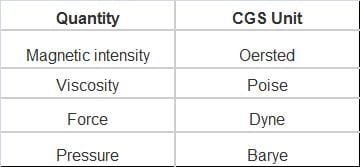RRB JE CBT 1 - Full Test 6 - Question 22

Where were the 2018 Summer Youth Olympic Games held?

Detailed Solution for RRB JE CBT 1 - Full Test 6 - Question 22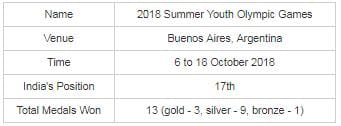RRB JE CBT 1 - Full Test 6 - Question 23

If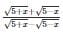= 3, then what is the value of x?

Detailed Solution for RRB JE CBT 1 - Full Test 6 - Question 23

Using Componendo and dividendo in the given equation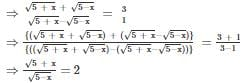Now squaring both sides

⇒ 5 + x = 20 - 4x

⇒ x = 3

∴ The value of x is 3

RRB JE CBT 1 - Full Test 6 - Question 24

There are three Kabaddi teams in a tournament A, B and C. If the difference between the average points of team A, B and B, C is 45, then what will be the difference of points between kabaddi team A and C?

Detailed Solution for RRB JE CBT 1 - Full Test 6 - Question 24

As we know average = sum of all the observation/total number of observation

Average of (A, B) – Average of (B, C) = 45

⇒ (A + B)/2 - (B + C)/2 = 45

⇒ A + B - B - C = 45 × 2

⇒ A - C = 90

∴ Difference between A and C = 90

RRB JE CBT 1 - Full Test 6 - Question 25

Who brokered the Indus Water Treaty between India and Pakistan?

Detailed Solution for RRB JE CBT 1 - Full Test 6 - Question 25
• World Bank brokered the Indus Waters Treaty between India and Pakistan.
• According to this agreement, control over the water flowing in three “eastern” rivers of India the Beas, the Ravi and the Sutlej was given to India, while control over the water flowing in three “western” rivers of India the Indus, the Chenab and the Jhelum was given to Pakistan.
RRB JE CBT 1 - Full Test 6 - Question 26

Which among the following is an example of connective tissue?

Detailed Solution for RRB JE CBT 1 - Full Test 6 - Question 26
• Bone is a type of dense connective tissue.
• Bones support and protect the various organs of the body, and provides structure and support for the body.
• Connective Tissues are used to bind and connect different tissues and organs.
• Skin is an example of an Epithelial tissue, Neurons is an example of a Nervous tissue and Rectum is an example of Muscular tissue.

RRB JE CBT 1 - Full Test 6 - Question 27

A boy starts from his house and walks at a speed of 5 km/hr and reaches his school 3 minutes late. Next day he starts at the same time and increases his speed by 4 km/hr and reaches 3 minutes early. What is the distance (in km) between the school and his house?

Detailed Solution for RRB JE CBT 1 - Full Test 6 - Question 27

Let the distance between the school and his house be ‘x’ km

Time taken on first day = Distance/speed = x/5 hrs.

His speed on next day = 5 + 4 = 9 km/hr.

Time taken on next day = x/9 hrs.

Now,

∵ He reached 3 min. late on the first day and reached 3 min. early the next day, this means that the difference between the time taken on the 2 days = 3 + 3 = 6 min. = 6/60 = 1/10 hrs.

⇒ x/5 – x/9 = 1/10

⇒ (9x – 5x)/45 = 1/10

⇒ 4x/45 = 1/10

⇒ x = 45/4 × 1/10

⇒ x = 1.125 km

∴ Distance between the school and his house is 1.125 km

RRB JE CBT 1 - Full Test 6 - Question 28

Ram celebrated his birthday on 3rd April 2000 on Monday. Next time in which year will he will celebrate his birthday on the same date and day?

Detailed Solution for RRB JE CBT 1 - Full Test 6 - Question 28

Number of odd days in 2000 = 1 (As 1st April comes after February)

Number of odd days from 2000 till 2001 = 1 + 1 = 2

Number of odd days from 2000 till 2002 = 2 + 1 = 3

Number of odd days from 2000 till 2003 = 3 + 1 = 4

Number of odd days from 2000 till 2004 = 4 + 2 = 6

Number of odd days from 2000 till 2005 = 6 + 1 = 7 = 0 odd days

Hence, Ram’s birthday on 3rd April 2006 will be on Monday.

RRB JE CBT 1 - Full Test 6 - Question 29

Development of 'Industry and Minerals' was on top priority in which of the following Five Year Plan?

Detailed Solution for RRB JE CBT 1 - Full Test 6 - Question 29

The Second Plan was particularly in the development of the public sector and "rapid Industrialisation". The plan followed the Mahalanobis model, an economic development model developed by the Indian statistician Prasanta Chandra Mahalanobis in 1953.

RRB JE CBT 1 - Full Test 6 - Question 30

A man is standing on a railway bridge, which is 70 m long. He finds that a train crosses the bridge in 5 and a half second, but crosses him in 2 seconds. Find the length of the train and its speed.

Detailed Solution for RRB JE CBT 1 - Full Test 6 - Question 30

⇒ Let the length of the train be x metres

⇒ Then, the train covers x metres in 2 seconds and (x + 70) m in 11/2 seconds

⇒ (x + 70)/x = 5.5/2

⇒ x = 40

⇒ Speed = 40/2 m/sec = (20 × 18/5) km/hr = 72 km/hr

RRB JE CBT 1 - Full Test 6 - Question 31

There are 52880 passengers who use Metro train. 49% of those use metro card and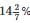of those who use metro card are males. What percentage of passengers who use metro card are females?

Detailed Solution for RRB JE CBT 1 - Full Test 6 - Question 31

Let the total passengers who use metro train be 100

So, number of passengers who use metro card = 100 × 49/100 = 49

now,= 1/7

So, number of male passengers who use metro card are males= 49 × 1/7 = 7

So, number of passengers who use metro card are females= 49 - 7 = 42

So, percentage of passengers who use metro card are females = 42.

RRB JE CBT 1 - Full Test 6 - Question 32

Directions: These questions are based on the following information. Study the information carefully and answer the questions.

The total population of a city is 57000. Out of this 85 % are literate. 45% of the total population are women. Out of the total literate population, men and women are in the ratio of 11 : 8 respectively.

Q. What is the total number of literate men and illiterate women?

Detailed Solution for RRB JE CBT 1 - Full Test 6 - Question 32

Total population of a city is 57000. Out of these 85% are literate.

∴ literate population = 57000 × 0.85 = 48450

⇒ Illiterate population = 57000 – 48450 = 8550

Out of the total literate population, men and women are in the ratio of 11 : 8 respectively.

∴ Literate women = 8/19 × 48450 = 20400

⇒ Illiterate women = 25650 – 20400 = 5250

⇒ literate men = 48450 – 20400 = 28050

Required number = 28050 + 5250 = 33300

RRB JE CBT 1 - Full Test 6 - Question 33

Which of the answer figures is the water image of the given figure?Detailed Solution for RRB JE CBT 1 - Full Test 6 - Question 33

In water image, only the top and bottom part of a given image are interchanged while left and right part remain the same.

Thus, we get the following water image: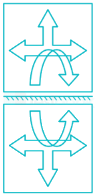Hence, the answer is figure 1.

RRB JE CBT 1 - Full Test 6 - Question 34

A merchant purchases a watch for Rs. 600 and fixes its marked price in such a way that after allowing a discount of 40%, he earns a profit of 20%. What is the marked price (in Rs.) of the watch?

Detailed Solution for RRB JE CBT 1 - Full Test 6 - Question 34

Let, the marked price = Rs. x

According to problem,

⇒ x - x × 40/100 = 600 + 600 × 20/100

⇒ 0.6x = 720

⇒ x = 1200

∴ Marked price of the watch = Rs. 1200

RRB JE CBT 1 - Full Test 6 - Question 35

By Selling 5 articles for 18rupee, a man losses 10%. If he sells 3 articles for Rs. 15, then what will be the profit percentage?

Detailed Solution for RRB JE CBT 1 - Full Test 6 - Question 35

Let, cost price of 5 article = Rs. x

Price at 10% loss = Rs. 18

According to problem,

⇒ x - x × 10/100 = 18

⇒ 0.9x = 18

⇒ x = 20

∴ Cost price of 5 article = Rs. 20

∴ Cost price of 3 article = Rs. 20 × 3/5 = Rs. 12

Selling price of 3 article = Rs. 15

∴ Profit percentage,

⇒15−1212×100%⇒15−1212×100%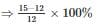⇒ 25%

RRB JE CBT 1 - Full Test 6 - Question 36

A steam engine converts the heat energy of steam into ______.

Detailed Solution for RRB JE CBT 1 - Full Test 6 - Question 36
• When water is heated under pressure, it results in the formation of steam.
• Steam engines utilize the energy of the steam generated to perform mechanical functions by using it to pusha piston which leads to motion.
RRB JE CBT 1 - Full Test 6 - Question 37

Directions: The question given below consists of a question and two Statements I and II given below it. You have to decide whether the data provided in the statements are sufficient to answer the questions. Read both the statements and give an answer.

On a TV channel four serials P, M, C and G were screened, one on each day, on four consecutive days but not necessarily in that order. On which day was serial C screened?
I. The first serial was screened on 23rd, Tuesday and was followed by serial G.
II. Serial P was not screened on 25th and one serial was screened between serials P and M.

Detailed Solution for RRB JE CBT 1 - Full Test 6 - Question 37

Given, four serials P, M, C and G were screened, one on each day, on four consecutive days.

From statement 1: The first serial was screened on 23rd, Tuesday and was followed by serial G.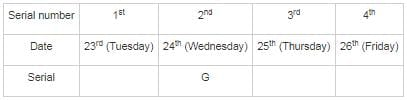We cannot conclude day of serial C.

From statement 2: Serial P was not screened on 25th and one serial was screened between serials P and M.

We can’t judge anything about C from it because nothing else is known as starting day or information about G or C or serial P and M are screened before 25th or after it etc.

On combining 1 and 2: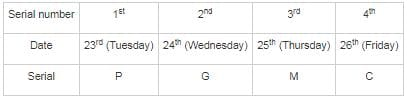From 1 and 2 clearly P and M fits only on 23rd and 25th date resp. and only 26th date is left for C Hence serial C is screened on 26th date on Friday.

Thus both the statements are required to answer the question.

RRB JE CBT 1 - Full Test 6 - Question 38

Which among the following is known as a Secondary Pollutant in the atmosphere?

Detailed Solution for RRB JE CBT 1 - Full Test 6 - Question 38
• A Primary Pollutant is a pollutant that emerges directly from any source whereas a secondary pollutant is formed when the primary pollutants react in the atmosphere.
• Ozone is an example of secondary pollutant since it is formed when nitrogen oxides and hydrocarbons combine in the presence of sunlight.
• Carbon monoxide is a primary pollutant.
RRB JE CBT 1 - Full Test 6 - Question 39

Which of the following is an output device?

Detailed Solution for RRB JE CBT 1 - Full Test 6 - Question 39
• An output device is any device used to send data from a computer to another device or user
• Printer - A device that accepts text and graphics output from a computer and transfers the information to paper, usually to standard size sheets of paper
• RAM (Random-access memory) - is a form of computer data storage that stores data and machine code currently being used
• ROM (Read-only memory) is a type of non-volatile memory used in computers and other electronic devices.
• Joystick an input device consisting of a stick that pivots on a base and reports its angle or direction to the device it is controlling.
RRB JE CBT 1 - Full Test 6 - Question 40

In the following question, select the odd letters from the given alternatives.

Detailed Solution for RRB JE CBT 1 - Full Test 6 - Question 40

The pattern followed here is,

R + 2 = T; T + 3 = W; W + 2 = Y;

G + 2 = I; I + 3 = L; L + 2 = N;

D + 2 = F; F + 3 = I; I + 2 = K;

L + 2 = N; N + 2 = P; P + 2 = R.

Hence, LNPR is the odd one out.

RRB JE CBT 1 - Full Test 6 - Question 41

The buccal cavity is a component of which organ system?

Detailed Solution for RRB JE CBT 1 - Full Test 6 - Question 41

Buccal cavity:

• a portion of the oral cavity bounded by the lips, cheeks, and gums
• is a component of the “digestive system” as the intake of food for digestion takes place through this
RRB JE CBT 1 - Full Test 6 - Question 42

The ratio of weekly incomes of A and B is 9 : 7 and the ratio of their expenditures is 4 : 3. If each saves Rs. 200 per week, then the sum of their weekly incomes is

Detailed Solution for RRB JE CBT 1 - Full Test 6 - Question 42

Given ratio of weekly income of A and B is 9 : 7 and ratio of their expenditure is 4 : 3.

Let the weekly income of A is Rs. 9x and weekly income of B be Rs. 7x

We know that, Expenditure = Income – Savings

Since savings of both A and B is Rs. 200. Thus,

Weekly expenditure of A = (9x – 200)

Similarly, weekly expenditure of B = (7x – 200)

Since the ratio of weekly expenditure is 4 : 3, hence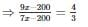⇒ 27x – 600 = 28x – 800

⇒ x = 200

Hence, the weekly income of A = 9 × x

= Rs. 1800

Also, weekly income of B = 7 × x

= Rs. 1400

∴ The sum of weekly income of A and B = Rs. (1800 + 1400)

= Rs. 3200

RRB JE CBT 1 - Full Test 6 - Question 43

In a certain code language, "STRANGE" is written as "HGIZMTV". How is "FRAUD" written in that code language?

Detailed Solution for RRB JE CBT 1 - Full Test 6 - Question 43

According to the given information: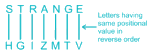Similarly,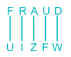Hence, "FRAUD" written in that code language as “UIZFW”.

RRB JE CBT 1 - Full Test 6 - Question 44

The numbers 3446, 5887 and 2445 are put side by side in the same order making a 100 digits number. Find the remainder when this 100 digit number is divided by 9.

Detailed Solution for RRB JE CBT 1 - Full Test 6 - Question 44

Since the number is of 100 digits and 3446, 5887 and 2445 are put side by side in the same order;

∴ 3446 must be repeated 9 times, 5887 & 2445 must be repeated 8 times;

∴ Sum of digits of 100 digits number = [3 + 4 + 4 + 6] × 9 + [5 + 8 + 8 + 7 + 2 + 4 + 4 + 5] × 8

⇒ 153 + 344

⇒ 497

Reminder when 497 is divided by 9 = 2

Hence, the remainder when the 100 digit number is divided by 9 would be 2.

RRB JE CBT 1 - Full Test 6 - Question 45

Calculate the value of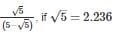Detailed Solution for RRB JE CBT 1 - Full Test 6 - Question 45

Given, √5 = 2.236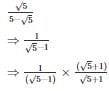⇒ (√5 + 1)/(5 - 1)

⇒ (2.236 + 1)/4

∴ 3.236/4 = 0.809

RRB JE CBT 1 - Full Test 6 - Question 46

Pointing to a photograph, Mira tells her husband, “This woman is the only daughter of your son’s maternal grandmother.”

How is Mira’s husband related to the woman in the photograph?

Detailed Solution for RRB JE CBT 1 - Full Test 6 - Question 46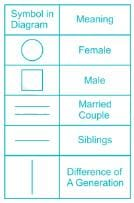Let us represent the given relations in the form of a family tree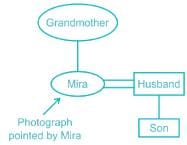Clearly, the woman in photograph is Mira.

Hence, husband is the correct answer.

RRB JE CBT 1 - Full Test 6 - Question 47

The bacillus that causes TB “Mycobacterium TB”  was discovered by ____________.

Detailed Solution for RRB JE CBT 1 - Full Test 6 - Question 47

Dr Robert Koch discovered Mycobacterium TB, the bacillus that causes TB. World Tuberculosis Day (TB) is observed every year on March 24 to commemorate Dr. Robert Koch’s announcement of the discovery.

RRB JE CBT 1 - Full Test 6 - Question 48

How many triangles are there in the given figure?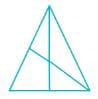Detailed Solution for RRB JE CBT 1 - Full Test 6 - Question 48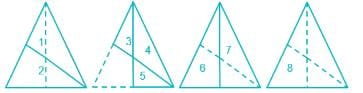Hence there are 8 triangles in the above figure.

RRB JE CBT 1 - Full Test 6 - Question 49

What is the sum of all natural numbers between 100 and 400 which are divisible by 13?

Detailed Solution for RRB JE CBT 1 - Full Test 6 - Question 49

The first and last number between 100 and 400 which are divisible by 13 are 104 and 390 respectively.

The numbers will be in arithmetic proportion because the common difference is 13, so the first term is 104 and the last term is 390.

So, Tn = a + (n – 1) × d

⇒ 390 = 104 + (n – 1) × 13

⇒ n = 23

⇒ Sn = n/2 × (a + l) = 23/2 × (104 + 390)

⇒ Sn = 5681

∴ The sum of all natural numbers between 100 and 400 which are divisible by 13 is 5681

RRB JE CBT 1 - Full Test 6 - Question 50

The blood vessel which supplies blood to the kidney is ________.

Detailed Solution for RRB JE CBT 1 - Full Test 6 - Question 50
• Renal artery is the branch of abdominal aorta that carries blood to kidney. It enters in the kidney through the hilum part.
• Pulmonary artery is the only artery that carries deoxygenated blood. It carries deoxygenated blood from right ventricle to lungs.
• Hepatic artery is a blood vessel that supplies oxygenated blood to the liver, duodenum, pancreas, and gallbladder.
• Carotid arteries are major blood vessels in the neck that supply blood to the brain, neck, and face.
RRB JE CBT 1 - Full Test 6 - Question 51

When the direction of motion of coil is perpendicular to the electromagnetic field, then the value of induced current is __________.

Detailed Solution for RRB JE CBT 1 - Full Test 6 - Question 51

A charged particle experiences no magnetic force when moving parallel to a magnetic field, but when it is moving perpendicular to the field it experiences a force perpendicular to both the field and the direction of motion.

RRB JE CBT 1 - Full Test 6 - Question 52

Identify the diagram that best represents the relationship among the given classes.
Prime Number, Even Number, Odd Number

Detailed Solution for RRB JE CBT 1 - Full Test 6 - Question 52

Prime Number, Even Number, Odd Number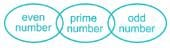2 is an even number which is also a prime number. Therefore prime numbers are both odd and even. From the given options, the above figure is the most closed relation between Prime Number, Even Number, Odd Number.

RRB JE CBT 1 - Full Test 6 - Question 53

During summer, water kept in an earthen pot becomes cool because of the phenomenon of ________.

Detailed Solution for RRB JE CBT 1 - Full Test 6 - Question 53
• In an earthen pot, water gets evaporated through the pores quickly and cooling is because of evaporation.
• For this, some heat energy is utilized and since this is taken from water, it leads to the lowering of water temperature.
RRB JE CBT 1 - Full Test 6 - Question 54

Ranganthittu Bird Sanctuary is located in which Indian state?

Detailed Solution for RRB JE CBT 1 - Full Test 6 - Question 54

Ranganathittu Bird Sanctuary is the largest bird sanctuary in Karnataka.

Asian openbill stork, Common spoonbill and Woolly-necked stork breed in this sanctuary regularly.

It lies on the banks of River Cauvery, this sanctuary is the home to some of the most exquisite birds.

Trick: A bird name Rangana flies toward Karnataka

RRB JE CBT 1 - Full Test 6 - Question 55

Directions: A statement is given followed by two inferences I and II. You have to consider the statement to be true even if it seems to be at variance from commonly known facts. You have to decide which of the given inferences, if any, follow from the given statement.

Statement: During Sheila Dikshit's tenure from 1998 to 2013, Delhi got an infrastructure boost with the metro chugging through the most congested neighborhoods, public transport switching to CNG and new flyovers adding to road space.

Inferences:
I: Before the construction of the metro, commuting through tight roads was very tough.
II: Before 1998, Delhi's infrastructure was not up to the mark.

Detailed Solution for RRB JE CBT 1 - Full Test 6 - Question 55

Metro has chugged though the tight neighbourhoods. So obviously it was hard to commute though them before metro. Also, the government must have spent a lot of money on this. Government won’t spend money on something which is already up to the mark. So the infrastructure must have been poor before 1998. Hence both the inferences follow.

RRB JE CBT 1 - Full Test 6 - Question 56

Contraction of heart is also known as-

Detailed Solution for RRB JE CBT 1 - Full Test 6 - Question 56

The rhythmic cycle of heart includes the contraction and relaxation of heart muscles to produce cardiac cycle. During this process contraction of heart muscle is known as systole and relaxation of heart muscle is known as diastole.

RRB JE CBT 1 - Full Test 6 - Question 57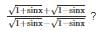Detailed Solution for RRB JE CBT 1 - Full Test 6 - Question 57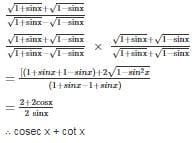RRB JE CBT 1 - Full Test 6 - Question 58

In a ΔPQR, I is a point which is equidistant from all the sides of the triangle. If ∠PRQ = 30°, then find ∠QIR + ∠PIR.

Detailed Solution for RRB JE CBT 1 - Full Test 6 - Question 58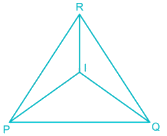Since I is equidistant from all the sides of the triangle, I must be incentre of the circle.

Given ∠PRQ = 30°

∴ ∠PIQ = 90 + 30/2 = 105°

∴ ∠QIR + ∠PIR = 360° – 105° = 255°

RRB JE CBT 1 - Full Test 6 - Question 59

A boy who is standing at the middle of the farm see a bird in the north an angle of elevation of 30° and after 1 minute he sees that bird at an angle of 60° in south direction. If that bird is flying at a height of 100√3 meters in straight-line then the speed of bird in Km./hr. is:

Detailed Solution for RRB JE CBT 1 - Full Test 6 - Question 59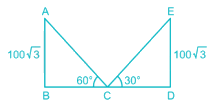In ΔABC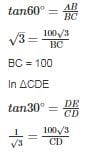CD = 300

Distance Covered = BC + CD

100 + 300 = 400 m.

Time = 60 second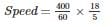Speed = 24

RRB JE CBT 1 - Full Test 6 - Question 60

Which answer figure will complete the pattern in the question figure? |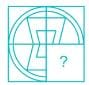Detailed Solution for RRB JE CBT 1 - Full Test 6 - Question 60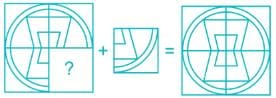Hence, option 4 will complete the given pattern.

RRB JE CBT 1 - Full Test 6 - Question 61

The Oxford Word of the Year 2018, originated from which of the following languages?

Detailed Solution for RRB JE CBT 1 - Full Test 6 - Question 61
• The Oxford Word of the Year 2018, originated from ‘Greek’.
• The Oxford Word of the Year 2018, was ‘toxic’, which means poisonous.
• ‘Toxic’ though is an English word but it was derived from Latin word ‘toxicum’, which in turn was taken from Greek word ‘toxikon pharmakon’.
RRB JE CBT 1 - Full Test 6 - Question 62

Who was the first person to reach the South Pole?

Detailed Solution for RRB JE CBT 1 - Full Test 6 - Question 62
• Norwegian explorer Roald Amundsen was the leader of the first expedition to reach the South Pole.
• He along with Helmer Hanssen, Oscar Wisting and Sverre Hassel reached the South Pole on 14th December 1911.
RRB JE CBT 1 - Full Test 6 - Question 63

The concept of Public Interest Litigation originated in which of the following countries?

Detailed Solution for RRB JE CBT 1 - Full Test 6 - Question 63
• The concept of Public Interest Litigation (PIL) originated in mid 1980s in the United States and the first legal aid office was established in New York in 1876.
• PIL is a litigation that is filed by the lawyers in the large interest of people.
• In India, an individual can file a PIL either in Supreme Court or High Court under the article 32 and 226 of the Indian constitution respectively.
RRB JE CBT 1 - Full Test 6 - Question 64

Pneumatic bone is an important characteristic of which of the vertebrate animals?

Detailed Solution for RRB JE CBT 1 - Full Test 6 - Question 64
• Pneumatic bone is an important characteristic of Aves.
• Present throughout the body of birds, pneumatic bones are hollow bones filled with air cells.
• This makes the skeleton light thereby enabling the birds to fly.
RRB JE CBT 1 - Full Test 6 - Question 65

Directions: The question below consists of a question and two statements numbered I and II given below it. You have to decide whether the data provided in the statements are sufficient to answer the question. Read both the statements and give an answer.

Among P, Q, R, S, and T sitting in a straight line, facing North, who sits exactly in the middle of the line?
I. P sits third to the left of S. T is an immediate neighbor of P as well as R.
II. T sits second to the left of S. Q is not an immediate neighbor of either T or S.

Detailed Solution for RRB JE CBT 1 - Full Test 6 - Question 65

From statement I:

• P sits third to left of S (2 spaces between P & S, with P on the left)
• T is an immediate neighbor of P as well as R (T surrounded by P & R)

Hence the following 2 cases arise (North is up for all diagrams):

Case I: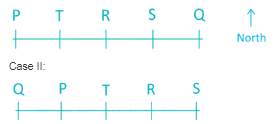Clearly we cannot determine exactly who is in the middle.

From statement II:

• T sits second to left of S (1 space between T & S, T being on the left)
• Q is not an immediate neighbor or either T or S (Q’s neighbor(s) are R and/or P)

Again the following four cases arise:

Case I & II: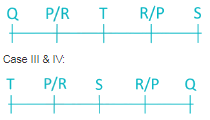Again we cannot determine whether S or T comes in the middle.

From the statement, I & II combined:

• P sits third to the left of S (2 spaces between P & S, with P on the left)
• T is an immediate neighbor of P as well as R (T surrounded by P & R)
• T sits second to left of S (1 space between T & S, T being on the left)
• Q is not an immediate neighbor of either T or S (Q’s neighbor(s) are R and/or P)

Only the following case satisfies all the conditions.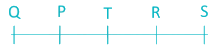Hence T occupies the middle position.

So the answer is ‘Data in both the Statement I and II together are necessary to answer the question.’

RRB JE CBT 1 - Full Test 6 - Question 66

A sum of money doubles itself in 6 years at a certain rate of compound interest. In how many years will be 16 times at the same rate of interest?

Detailed Solution for RRB JE CBT 1 - Full Test 6 - Question 66

Let the sum be ‘x’ and the rate be ‘r’

∴ According to the 1st given condition,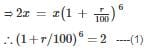For the 2ndcondition, Let t be the number of years required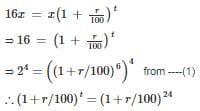∴ t = 24

∴ The amount will become 16 times in 24 years.

RRB JE CBT 1 - Full Test 6 - Question 67

A dishonest shopkeeper cheats at time of buying and selling the products. He weights 20% more at time of buying and 10% less at time of selling. What is his total profit?

Detailed Solution for RRB JE CBT 1 - Full Test 6 - Question 67

Given that he cheats 20% at time of buying

⇒ Goods bought = 1000(1 + 20/100)

⇒ 1200 g at price of 1000 g

Given that he cheats 10% at time of buying

⇒ Goods sold = 1000(1 – 10/100)

⇒ 900g at price of 1000 g

Since cost price = selling price

⇒ Profit percentage = goods left/goods sold × 100

⇒ [(1200 – 900)/900] × 100%

⇒ [300/900] × 100%

⇒ [1/3] × 100%

⇒ 33.33%

∴ Profit percentage = 33.33%

RRB JE CBT 1 - Full Test 6 - Question 68

How does calcium carbide help in the ripening of mangoes?

Detailed Solution for RRB JE CBT 1 - Full Test 6 - Question 68
• Calcium carbide reacts with the moisture emanated by the respiration of the fruit.
• It liberates a gas called acetylene which helps in ripening the fruit.
RRB JE CBT 1 - Full Test 6 - Question 69

In the figure, ABCD is a square. Find the length of tangent PC, if the radius of the circle is 5 cm.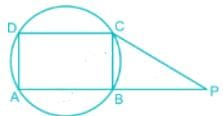Detailed Solution for RRB JE CBT 1 - Full Test 6 - Question 69

Radius of the circle is 5 cm

∴ Side of the square AB = BC = 5√2 cm

Suppose PB = x cm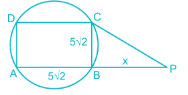In ΔPBC

⇒ PC2 = [(5√2)2 + x2]

We know that∶

PC2 = PB × PA

⇒ [(5√2)2 + x2] = x × (x + 5√2)

⇒ 50 + x2 = x2 + 5√2x

⇒ x = 5√2 cm

∴ PC = √[(5√2)2 + x2] = √(50 + 50) = 10 cm

Previous

RRB JE CBT 1 - Full Test 6 - Question 70

In the following question, select the missing number from the given series.
9, 16, 13, 12, 17, 8, ?, ?

Detailed Solution for RRB JE CBT 1 - Full Test 6 - Question 70

This series is combination of two series which are placed alternately,

Series I : 9, 13, 17, ?

9 + 4 = 13

13 + 4 = 17

17 + 4 = 21

Series II : 16, 12, 8, ?

16 – 4 = 12

12 – 4 = 8

8 – 4 = 4

Hence, 21, 4 is the correct answer.

RRB JE CBT 1 - Full Test 6 - Question 71

Why does Table salt become moist during the rainy season?

Detailed Solution for RRB JE CBT 1 - Full Test 6 - Question 71

Table salt becomes moist during the rainy season because sodium chloride contains impurities like magnesium chloride which absorb water and makes it damp.

RRB JE CBT 1 - Full Test 6 - Question 72

In the circuit shown in the figure, the voltmeter reading would be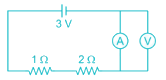Detailed Solution for RRB JE CBT 1 - Full Test 6 - Question 72

The voltmeter is connected in parallel with an ammeter

The ideal resistance of ammeter is zero, Hence voltage drop across it (IR=0)

RRB JE CBT 1 - Full Test 6 - Question 73

Who is the author of a book named "Worshipping False Gods"?

Detailed Solution for RRB JE CBT 1 - Full Test 6 - Question 73
• Arun Shourie is the author of the book "Worshipping False Gods".
• Arun Shourie is a journalist, economist, author and politician.
• He has worked as an economist, consultant, and editor at World Bank, Planning Commission of India, Indian Express and Times of India respectively.
• He was also appointed as Minister of Communications and Information Technology in 1998 by Shri Atal Bihari Vajpayee.
• This book is regarding critical look at the legend B.R. Ambedkar.
RRB JE CBT 1 - Full Test 6 - Question 74

In the nth term of an A.P. be (2n - 1), then the sum of its first n terms will be

Detailed Solution for RRB JE CBT 1 - Full Test 6 - Question 74

Let ‘a’ be the first term and ‘d’ the common difference.

Now, sum of first n numbers of an AP =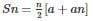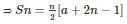Finding, the AP for different values of ‘n’

n = 1: a1 = 2 × 1 – 1 = 2 -1 = 1

n = 2: a2 = 2 × 2 – 1 = 4 – 1 = 3

∴ d = 3 – 1 = 2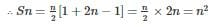RRB JE CBT 1 - Full Test 6 - Question 75

What is the unit digit of 7139?

Detailed Solution for RRB JE CBT 1 - Full Test 6 - Question 75

(7139) = (7136 + 3) = (7(4 × 34 + 3)) = 74 × 34 × 73

We know that cyclicity of 7 is 4 only, so (74 × 34) will have the unit digit 1

⇒ Unit digit of (7139) = Unit digit of (1 × 73) = Unit digit of 343

∴ Unit digit of (7139) = 3

RRB JE CBT 1 - Full Test 6 - Question 76

In case CO2 of earth’s atmosphere disappears, the temperature of the earth’s surface would

Detailed Solution for RRB JE CBT 1 - Full Test 6 - Question 76

Carbon dioxide gas is crucial for the maintenance of life-sustaining temperature on Earth due to its property of trapping thermal solar radiation. The mechanism by which carbon dioxide traps heat in the atmosphere is commonly referred to as the "greenhouse effect", in absence of which Earth’s temperature would drop drastically.

RRB JE CBT 1 - Full Test 6 - Question 77

Ramsar convention took place in which year?

Detailed Solution for RRB JE CBT 1 - Full Test 6 - Question 77

Ramsar convention is an international treaty for the conservation and sustainable utilization of wetlands, recognizing the fundamental ecological functions of wetlands. It is named after the city of Ramsar in Iran, where the convention was signed in 1971.

RRB JE CBT 1 - Full Test 6 - Question 78

Directions: The question below consists of a question and two statements numbered I and II given below it. You have to decide whether the data provided in the statements are sufficient to answer the question. Read both the statements and give the answer.

Who amongst X, Z, B, D, and F was the first to reach the party hall?
I. Z, who reached earlier than F, X and B was not the first to reach.
II. X, who reached earlier than F and B could not reach earlier than D, who was at the party hall before Z.

Detailed Solution for RRB JE CBT 1 - Full Test 6 - Question 78

Note: Here the person after ‘→’ is late one.

From statement 1: Z, who reached earlier than F, X and B was not the first to reach.

⇒ Z → F, X and B  was not first to reach and only D is left,

so D reached the party first.

Hence, Data in statement I alone is sufficient to answer the question

From statement 2: X, who reached earlier than F and B could not reach earlier than D, who was at the party hall before Z.

⇒ X → F, B and D → X & D → Z

⇒ D → X → F, B & D → Z

Clearly, Data in statement II alone are sufficient to answer the question

from statements II we can depict that D is the first to reach the party hall.

Hence, Data in statement I alone or in statement II alone is sufficient to answer the question.

RRB JE CBT 1 - Full Test 6 - Question 79

Bernoulli’s theorem is applicable only for ________.

Detailed Solution for RRB JE CBT 1 - Full Test 6 - Question 79

In fluid dynamics, Bernoulli’s theorem gives the relation among the elevation, velocity, and pressure in a moving fluid, for which, the viscosity and compressibility are negligible and the flow is laminar or steady. The theorem is applicable for incompressible flows.

RRB JE CBT 1 - Full Test 6 - Question 80

The Islets of Langerhans is a part of which among the following in the human body?

Detailed Solution for RRB JE CBT 1 - Full Test 6 - Question 80
• The Islets of Langerhans is the part of the pancreas in the human body.
• It was discovered by the medical scientist Langerhans.
• Hormones such as insulin, glucagon and somatostaintin are secreted from this part of the human body.
• That is why malfunctioning of the pancreas will cause diabetes in the human body.
RRB JE CBT 1 - Full Test 6 - Question 81

Which transitions are studied by UV Spectrometer?

Detailed Solution for RRB JE CBT 1 - Full Test 6 - Question 81

Ultra Violet Spectrometer is basically used to study electronic transitions.

• It is mainly used for the measurement of reflectance of transparent solids and solutions as well as the intensity of a light beam.
RRB JE CBT 1 - Full Test 6 - Question 82

In the given letter series, which one set of letters when sequentially placed in the gaps shall complete it?
p _ n n m _ p m n _ m p _ m n _ m p

Detailed Solution for RRB JE CBT 1 - Full Test 6 - Question 82

p _ n n m _ p m n _ m p _ m n _ m p

1. n m n p p ⇒ p nnn m m p m n n m p p m n p m p

2.m n p n p ⇒ p m n n m n p m n p m p n m n p m p

3.m p n p n ⇒ p m n/n m p/ p m n/n m p/p m n/n m p

4.n p m p n ⇒ p nnn m pp m n m m p p m n n m p

Here in option 3) we can have repeated pattern of “pmn” and ‘’nmp’’alternatively.

RRB JE CBT 1 - Full Test 6 - Question 83

If a long hollow copper pipe carries a direct current, the magnetic field associated with the current will be _________

Detailed Solution for RRB JE CBT 1 - Full Test 6 - Question 83

Inside the conductor

Selecting any closed loop path inside the hollow conductor

I = 0

From Amperes Circuital law

∫H.dl=0

H = 0

B = 0

Outside the conductor, selecting a closed path, there is currently associated

Hence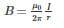RRB JE CBT 1 - Full Test 6 - Question 84

What is the positive value of m for which the roots of the equation 12x2 + mx + 5 = 0 are in the ratio 3 ∶ 2?

Detailed Solution for RRB JE CBT 1 - Full Test 6 - Question 84

Let the roots be 3t and 2t respectively

Product of the roots = c/a

3t × 2t = 5/12

t2 = 5/72

∴ t = ± √5/(6√2)

Sum of roots = -b/a

3t + 2t = -m/12

-60t = m

∴ m = -60 × ± √5/(6√2) = ± 5√10

Only positive value of m is required

∴ m = 5√10

RRB JE CBT 1 - Full Test 6 - Question 85

Saira, Divanshi, Prahil, Kashvi, Naira, and Devina are cousins. They all like different T.V. Shows- Kumkum Bhagya, Bigg Boss, Ye Hai Mohabbatein, Naagin, Bepannah, and Ishqbaaz. Devanshi likes watching Ye Hai Mohabbatein. Neither kashvi nor Prahil likes Bepannah and Bigg Boss. Saira likes Kumkum Bhagya. Neither Naira nor Devina like Ishqbaaz and Naagin. Kashvi likes Naagin and Devina likes Bepanah. Who among the following likes watching Bigg Boss?

Detailed Solution for RRB JE CBT 1 - Full Test 6 - Question 85

Cousins: Saira, Divanshi, Prahil, Kashvi, Naira and Devina.

T.V. Show: Kumkum Bhagya, Bigg Boss, Ye Hai Mohabbatein, Naagin, Bepannah and Ishqbaaz.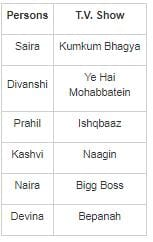Hence, Naira likes Bigg Boss.

RRB JE CBT 1 - Full Test 6 - Question 86

Which disease is caused by the pollution of cadmium metal?

Detailed Solution for RRB JE CBT 1 - Full Test 6 - Question 86

Itai-Itai disease name was given to the mass cadmium poisoning of Toyama Prefecture, Japan, starting around 1912. This disease was caused due to allergic pollution of cadmium metal.

RRB JE CBT 1 - Full Test 6 - Question 87

If the roots of the equation x3 – 12x2 + 39x – 28 = 0 are in A.P., then their common difference will be;

Detailed Solution for RRB JE CBT 1 - Full Test 6 - Question 87

Roots of Given equation x3 – 12x2 + 39x – 28 = 0 are in A.P.

Let α – β, α, α + β be the roots of the equation.

Here β is the common difference

Sum of the roots = α – β + α + α + β = -b/a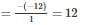3α = 12 ⇒ α = 4

and product  = - d/a

(α – β) α(α + β) = 28

α = 4

(4 – β) 4(4+ β) = 28

(4 – β) (4+ β) = 28/4 = 7

16 -  β2 = 7

⇒ β = ± 3

RRB JE CBT 1 - Full Test 6 - Question 88

The ‘Alps Mountains’ are which type of mountains?

Detailed Solution for RRB JE CBT 1 - Full Test 6 - Question 88
• The Alps Mountains are ‘Young Fold Mountains’.
• The Alps Mountains are the highest and most extensive mountain range in Europe which were formed millions of years ago.
• The effects of folding on the layers within the upper part of the crust of Earth leads to the formation of the ‘fold mountains.
RRB JE CBT 1 - Full Test 6 - Question 89

In the following question, select the number which can be placed at the sign of question mark (?) from the given alternatives.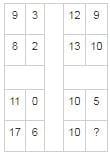Detailed Solution for RRB JE CBT 1 - Full Test 6 - Question 89

The pattern followed in each matrix is as follows,

In matrix 1 → 8 - 9 + 3 = - 1 + 3 = 2

In matrix 2 → 13 - 12 + 9 = 1 + 9 = 10

In matrix 3 → 17 - 11 + 0 = 6 + 0 = 6

Similarly,

Inn matrix 4 → 10 - 10 + 5 = 5

Hence, the correct answer is 5.

RRB JE CBT 1 - Full Test 6 - Question 90

Integrated Circuits are made up of which of the following?

Detailed Solution for RRB JE CBT 1 - Full Test 6 - Question 90
• An IC or Integrated Circuit, also known as microchip, chip, or microelectronic circuit is an assembly of electronic components which are fabricated as a single unit.
• The active and passive devices such as transistors and resistors and their interconnections are built on a thin substrate of silicon, a semiconductor material.
RRB JE CBT 1 - Full Test 6 - Question 91

First battle of Panipat was fought in the year ________.

Detailed Solution for RRB JE CBT 1 - Full Test 6 - Question 91

The First Battle of Panipat was fought on 21 April 1526 between the forces of Babur and Ibrahim Lodhi in which Lodhi was defeated and the Mughal rule was established in India.

RRB JE CBT 1 - Full Test 6 - Question 92

Which of these metals are not present in German silver?

Detailed Solution for RRB JE CBT 1 - Full Test 6 - Question 92
• German silver is a copper alloy with nickel and zinc.
• Silver is not present in it. The usual formulation is 60% copper, 20% nickel and 20% zinc. It is also known as nickel silver.
• It was originally named for its silver-white colour.
RRB JE CBT 1 - Full Test 6 - Question 93

A bifocal lens is used as a remedy in which of the following defects in the eyes?

Detailed Solution for RRB JE CBT 1 - Full Test 6 - Question 93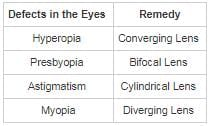RRB JE CBT 1 - Full Test 6 - Question 94

Which country has allowed India to ferry Haj pilgrims via sea route?

Detailed Solution for RRB JE CBT 1 - Full Test 6 - Question 94
• Due to good ties with India, the Saudi Arabian administration has allowed Haj Pilgrims to enter the country via sea route.
• This was previously banned by the country.
RRB JE CBT 1 - Full Test 6 - Question 95

Of the 5 subjects, the average marks of Rakesh in first 3 Subjects was 79 and the average marks in the last 3 subjects was 86. If his marks in the third subject were 80, then what were his average marks in all the 5 subjects?

Detailed Solution for RRB JE CBT 1 - Full Test 6 - Question 95

We know,

Average = sum of all observations/number of observations

⇒ Average marks of Rakesh in first 3 subjects = 79

⇒ Sum of marks in first 3 subjects = 79 × 3 = 237

Also,

Average marks of Rakesh in last 3 subjects = 86

⇒ Sum of marks in last 3 subjects = 86 × 3 = 258

Marks in third subject = 80

Sum of marks in all the five subjects = 237 + 258 - 80 = 415

∴ Average of all the five subjects = 415/5 = 83

RRB JE CBT 1 - Full Test 6 - Question 96

A man sells two articles. The cost price of the first article is equal to the selling price of the second article. The first article is sold at a profit of 20% and the second articles is sold at a loss of 20%. What is the ratio of the selling price of the first article and the cost price of the second article?

Detailed Solution for RRB JE CBT 1 - Full Test 6 - Question 96

Let the cost price of the first article be Rs. ‘x’

But, the first article is sold at a profit of 20%,

⇒ Selling price of first article = x + 20% of x = x + x/5 = (6/5)x

Now,

Selling price of second article = Cost price of first article = Rs. x

But, the second article is sold at a loss of 20%,

⇒ (100 - 20)% of Cost price of second article = Selling price

⇒ 80% of Cost price of second article = Selling price

⇒ (4/5) × Cost price of second article = x

⇒ Cost price of second article = (5/4)x

∴ Required ratio = (6x/5) ∶ (5x/4) = 24 ∶ 25

RRB JE CBT 1 - Full Test 6 - Question 97

Which of the following is the largest inland saltwater of India?

Detailed Solution for RRB JE CBT 1 - Full Test 6 - Question 97

Rajasthan’s Sambhar Lake is the largest inland salt water lake of India with the surface area of approximately 200 sq. kilometers.

• It is located 96 km southwest of the city of Jaipur (Northwest India) and 64 km northeast of Ajmer along National Highway 8 in Rajasthan.
• It surrounds the historical Sambhar Lake Town.
RRB JE CBT 1 - Full Test 6 - Question 98

In the following question, a group of interrelated figures is given. Choose a figure from the given alternatives, which is similar to the given figures and hence belongs to the same group.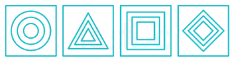Detailed Solution for RRB JE CBT 1 - Full Test 6 - Question 98

In the given series all three shapes in each figure are the same.

The only figure which belongs to the same group is option 3.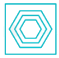Hence, the answer is option 3.

RRB JE CBT 1 - Full Test 6 - Question 99

Directions: One statement is given, followed by two conclusions, I and II. You have to consider the statement to be true, even if it seems to be at variance from commonly known facts. You are to decide which of the given conclusions be definitely drawn from the given statement.

Statement: All luxury hotels have a well maintained swimming pool. People tend to get attracted to the facilities provided by the hotels.

Conclusions:
I.
Accommodation in luxuries hotels are very limited and reserved.
II. Sophisticated facilities are important elements for luxury hotels.

Detailed Solution for RRB JE CBT 1 - Full Test 6 - Question 99

Solution: The statement states that luxury hotels have facilities which attract public attention.

These facilities are important elements in successful functioning of those hotels. Thus conclusion II follows. We cannot conclude that luxury hotels have limited accommodation and reserved entry from the statement. It changes with the hotels policy and capabilities. Hence only conclusion II is true to the statement.

RRB JE CBT 1 - Full Test 6 - Question 100

The policy of the Doctrine of lapse was introduced by ________.

Detailed Solution for RRB JE CBT 1 - Full Test 6 - Question 100
• The policy of Doctrine of lapse was introduced by Lord Dalhousie.
• According to "Doctrine of Lapse" when the ruler of a protected state dies without a natural heir, his state would be annexed by British India instead of passing it to an adopted heir.
• The states that were annexed by the policy of Doctrine of Lapse were Satara in 1848, Jaipur in 1849 and Bhagat in 1850 among others.
• Lord Dalhousie came to India as the Governor-General in 1848.

## RRB JE (Railways Junior Engineer) CBT Mock Test Series

19 tests
Information about RRB JE CBT 1 - Full Test 6 Page
In this test you can find the Exam questions for RRB JE CBT 1 - Full Test 6 solved & explained in the simplest way possible. Besides giving Questions and answers for RRB JE CBT 1 - Full Test 6, EduRev gives you an ample number of Online tests for practice

## RRB JE (Railways Junior Engineer) CBT Mock Test Series

19 tests(Scan QR code)